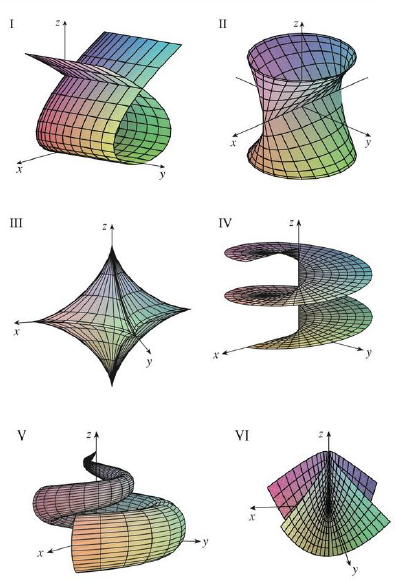Chapter 16.6, Problem 13E

Chapter
Section
Textbook Problem

Match the equations with the graphs labeled I–VI and give reasons for your answers. Determine which families of grid curves have u constant and which have v constant.13. r(u, v) = u cos v i + u sin v j + v kTo determine

To match: The equation r(u,v)=ucosvi+usinvj+vk with the graphs labeled I-VI in the textbook and to explain the reason for the combination.

Explanation

Given data:

The given vector equation as follows.

r(u,v)=ucosvi+usinvj+vk

Write the vector equation as follows.

r(u,v)=ucosvi+usinvj+vk

Write the parametric equations from the vector equation as follows.

x=ucosv,y=usinv,z=v (1)

Determination of grid curves when u is constant:

If the parameter u is held constant in the equation (1), the projection onto the xy-plane is circular with z=v .

Thus, each grid curve is a helix when the parameter u is constant

Still sussing out bartleby?

Check out a sample textbook solution.

See a sample solution

The Solution to Your Study Problems

Bartleby provides explanations to thousands of textbook problems written by our experts, many with advanced degrees!

Get Started

A sample with a mean of M = 8 has X = 56. How many scores are in the sample?

Essentials of Statistics for The Behavioral Sciences (MindTap Course List)

II. Operating Leverage and Business Risk Once you have determined the break-even poi...

Mathematical Applications for the Management, Life, and Social Sciences

Multiply: (+5)(7)

Elementary Technical Mathematics

The third partial sum of is:

Study Guide for Stewart's Multivariable Calculus, 8th